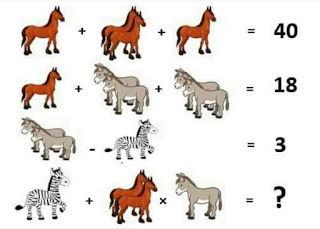# Horse Donkey Zebra Math Puzzle - with Answer### Can you solve the Horse Donkey Zebra Math Puzzle?Find the values of Horse, Donkey & Zebra and Solve the last equation.

The correct answer is given below.

ANSWER

41 is the answer

Explanation
Equation 1: 10 + (10 + 10) + 10 = 40  (So, the value of Horse = 10)
Equation 2: 10 + (2 + 2) + (2 + 2) = 18  (So, the value of 1 Donkey = 2)
Equation 3: (2 + 2) - 1 = 3   (So the value of Zebra = 1)
Equation 4: 1 + (10 + 10) x 2
Applying BODMAS rule:
=> 1 + (20 x 2)
=> 1 + 40
=> 41

This post first appeared on WhatsApp Puzzles With Answers, please read the originial post: here

# Share the post

Horse Donkey Zebra Math Puzzle - with Answer

×

# Subscribe to Whatsapp Puzzles With Answers

Get updates delivered right to your inbox!

×